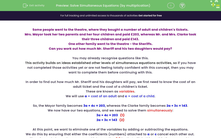# Solve Simultaneous Equations (by multiplication)

In this worksheet, students will learn how to solve simultaneous equations using the elimination method, in which both equations needs to be multiplied. They will also solve contextualised question based on simultaneous equations.Key stage:  KS 4

Year:  GCSE

GCSE Subjects:   Maths

GCSE Boards:   Pearson Edexcel, OCR, Eduqas, AQA,

Curriculum topic:   Algebra

Curriculum subtopic:   Solving Equations and Inequalities Algebraic Equations

Difficulty level:#### Worksheet Overview

Some people went to the theatre, where they bought a number of adult and children's tickets.

Mrs. Mayor took her two parents and her four children and paid £203, whereas Mr. and Mrs. Clarke took their three children and paid £143.

One other family went to the theatre - the Sheriffs.

Can you work out how much Mr. Sheriff and his two daughters would pay?

You may already recognise questions like this.

This activity builds on ideas established other levels of simultaneous equations activities, so if you have not completed those activities yet or are not feeling totally confident with this concept, then you may want to complete them before continuing with this.

In order to find out how much Mr. Sheriff and his daughters will pay, we first need to know the cost of an adult ticket and the cost of a children's ticket.

These are known as variables.

We will use = cost of an adult and c = cost of a child.

So, the Mayor family becomes 3a + 4c = 203, whereas the Clarke family becomes 2a + 3c = 143.

We now have our two equations, and we need to solve them simultaneously:

3a + 4c = 203   (1)

2a + 3c = 143   (2)

At this point, we want to eliminate one of the variables by adding or subtracting the equations.

We do this by ensuring that either the coefficients (numbers) attached to a or c cancel each other out.

There is no whole number we can multiply equation (2) by that will do this, so this time we need to multiply equation (1) by one number and equation (2) by a different number to achieve this.

If we want to equate the coefficient of c, we can multiply (1) by 3 and (2) by 4 which will give us 12c in both:

9a + 12c = 609   (3)

8a + 12c = 572   (4)

Since both equations have been changed we label the new equations (3) and (4).

Now we have the same number of a's in both equations and the signs in front are the same so we can subtract (4) from (3):

9a + 12c = 609   (3)

8a + 12c = 572   (4)

a = 37   (3) - (4)

So, an adult ticket costs £37.

Now, to find the cost of a child's ticket, substitute a = 37 into any of the four equations:

2 x 37 + 3c = 143  [substitute into (2)]

74 + 3c = 143

3c = 69

c = 23

So, a child's ticket costs £23.

Now we can find the cost for the Sheriff family which is one adult and two children:

37 + 2 x 23 = 83 so they would pay £83.

If you now compare what we have done here with solving simultaneous equations by elimination, you will see that in this example we multiplied both equations in order to equate the coefficients of one variable.

Other than that, everything else is the same.

We could have equally multiplied equation (1) by 2 and equation (2) by 3 which would have given us 6a in both and then we could have eliminated a.

This method would have been equally valid and enabled us to reach the correct answer too.

Now, let's look at another pair of simultaneous equations.

This time we will use just the equations without the real-life problem.

Solve 2x + 5y = -2  (1)

and 3x - 4y = 20  (2)

To equate coefficients of y, we need to multiply (1) by 4 and (2) by 5:

2x + 5y = -2  (1) × 4

3x - 4y = 20  (2) × 5

8x + 20y = -8  (3)

15x - 20y = 100  (4)

Since the signs in front of the y's are different, we need to add equations (3) and (4):

8x + 20y = -8  (3)

15x - 20y = 100  (4)

23x =  92   (3) + (4)

x = 4  [divide by 23]

2 x 4 + 5y = -2  [substitute into (1)]

8 + 5y = -2

5y = -10  [subtract 8]

y = -2   [divide by 5]

3 x 4 - 4 x -2 = 20  [check in (2)]

12 - -8  = 20 [correct]

So x = 4 and y = -2.

Hopefully now you are feeling ready to solve some simultaneous equations of your own.

As these methods are long and complex, you will want to have a pen and paper handy to record your working.

Remember we are looking for a way to eliminate one of the coefficients, so just look for the best numbers to multiply each equation by so they match.

### What is EdPlace?

We're your National Curriculum aligned online education content provider helping each child succeed in English, maths and science from year 1 to GCSE. With an EdPlace account you’ll be able to track and measure progress, helping each child achieve their best. We build confidence and attainment by personalising each child’s learning at a level that suits them.

Get started